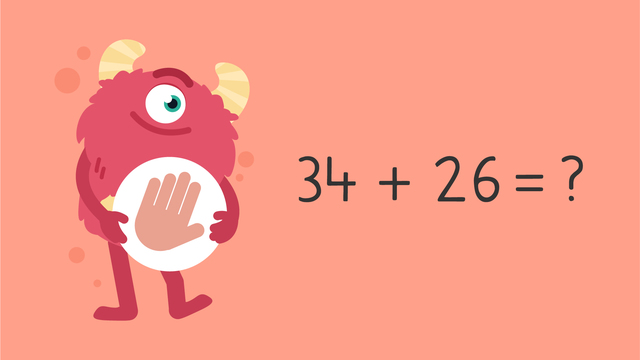# Make a Number Line to Add — Let's Practice!Rating

Ø 5.0 / 2 ratings
The authorsTeam Digital

## Make a Number Line to Add — Let's Practice! exercise

Would you like to apply the knowledge you’ve learned? You can review and practice it with the tasks for the video Make a Number Line to Add — Let's Practice!.
• ### Show the steps of using a number line to solve a problem.

Hints

Try making your own number line to solve 17 + 22. What did you do first?

Think about the problem step by step. Before we count forward by the second addend, what do we need to do?

Solution

The steps to using a number line to solve a problem are:

1. Draw a number line.
2. Put the larger addend on the left.
3. Count forward by small amounts from the second addend.
4. Solve the equation.

• ### Show how to add using the number line.

Hints

Remember, put the larger addend on the left.

Think about the gaps at the top, that is where we move forward with small amounts of the second addend. The gaps start with + __.

The addends are filled in. Place the sums below on the number line.

Solution

This is how we use a number line to add!

The larger addend 76 is on the left. The smaller addend 16 is split into small amounts (+10 and +6).

76 + 16 = 92.

• ### Use the number line to add the numbers and find the answer.

Hints

Remember, first we put the larger addend on the left. Which number is larger?

Once the larger addend is on the left, count forward by small amounts of the second addend. Fill in the blanks as you count on.

Solution

53 + 32 = 85.

We start at the larger addend, 53. From there, we add the smaller addend 32 by small amounts (10 + 10 + 10 + 2) until the numbers are added to make 85.

• ### Use the number line to solve the equation.

Hints

Remember, put the larger addend on the left.

To fill in the numbers on the top, think about how to split up the number 12 into smaller amounts.

The addends are filled in on the number line. Can you place the sums below?

Solution

64 + 12 = 76.

First, we put the larger addend 64 on the left. Then, we split 12 into smaller amounts (10 + 2). 64 + 10 = 74, 74 + 2 = 76.

• ### Complete the number line.

Hints

Add each number (10 and 7) to fully add 17. Use the number line to guide you.

Solution

The number line starts with the larger addend (40) on the left. Next, we count forward by small amounts of the second addend: 40 +10 = 50, 50 + 7 = 57.

The answer to 40 + 17 = 57

• ### Create your own number lines to solve.

Hints

Remember to break the second addend into small amounts. For example: 24 + 14. 14 is the smaller addend. We can break it into +10 and +4.

Remember, you have solved the problem once you have added the entire second addend.

Solution

By making our own number lines, we can solve the equations:

24 + 14 = 38

45 + 18 = 63

58 + 13 = 71